2017-04-12 10:43:12 J10527 阅读数 2742https://github.com/jah10527/rot3d

2018-11-12 11:37:18 YuYunTan 阅读数 453

# 李群与李代数大全（1） 在计算机视觉应用详细描述

本次博文要讲述的是在上一次博文中没有完全展开描述的李群和李代数，可以参考博主这篇博文，大致了解一下李群和李代数以及刚体运动，算算本博文的简洁精炼版本，本博文的系列对其进行详细补充和描述，并且使得可以对李代数类似于字典一般便于查找。

博主只是进行了大致浏览和概括，但是完全没有达到博主的期望，现在博主大刀阔斧的再次操刀，要进行一次最为齐全的李群李代数的讲解。

本次的博文会十分的长，会包括部分公式的推导，用于处理表示2D和3D空间变换的李群。

本博文主要是介绍关于李群和李代数在计算机视觉中的应用，原先博文的李群李代数为此博文的简化版本。后面系列的博文就讲纯粹的李群李代数理论及其推算以及相应的公式。

首先我们要知道，李群是一个拓扑群，也是一个光滑的流形，具有一些其他不错的属性。 与每个李群相关联的是李代数，它是下面讨论的向量空间。 重要的是，李群及其李代数密切相关，允许将一个中的计算有效地映射到另一个中【或者相互转换，这点在我写过的博文指出过，指数映射和对数，这是一对反函数】。

我们给出一些略微的李群总结

$SO(3)$ 3D旋转 3 3D旋转矩阵
$SE(3)$ 3D刚性变换 6 在齐次4维矢量上线性变换
$SO(2)$ 2D旋转 1 2D旋转矩阵
$SE(2)$ 2D刚性变换 3 在齐次3维矢量上线性变换
$Sim(3)$ 3D相似变换

7 在齐次4维矢量上线性变换

## 为什么说李群可以使用在机器人学和计算机视觉中

机器人学和计算机视觉中的许多问题涉及3D几何中的操纵和估计。 如果没有一个连贯而强大的框架来表示和处理3D转换，这些任务繁重而且非常危险。 必须对变换进行组合，反转，分解和插值。 李群及其相关机制解决了所有这些操作，并以条理化方式进行，因此这样的事实一旦形成，就可以令人信服。

## 三维欧氏空间

我们将使用 $\mathbb{E}^3$ 来表示熟悉的三维欧氏空间。 一般来说，欧氏空间是一个元素，其元素满足欧氏的五个公理。 在分析上，三维欧氏空间完全可以用笛卡尔坐标系表示：每个点$\rho\in \mathbb{E}^3$可以用$\mathbb{R}^3$中的一个点用三个坐标来识别

$X \dot= [x_1,x_2,x_3]^T = \begin{bmatrix}x_1\\x_2\\x_3\end{bmatrix}\in \mathbb{R}^3$

通过这种形式使得我们可以知道，在笛卡尔坐标下，$\mathbb{E}^3$$\mathbb{R}^3$是一一对应关系，这样可以假设在谈论点和其坐标，二者是等价的。

笛卡尔坐标是使测量距离和角度成为可能。 为此，必须为$\mathbb{E}^3$ 赋予度量标准。 度量的精确定义依赖于向量的概念。

### 向量

在欧氏空间中，向量$v$由一对点$p,q\in \mathbb{E}^3$确定，并且被定义为连接p到q的有向箭头，表示为$v=\overrightarrow{pq}$

点$p$通常被称为向量$v$的基点(base point)。在坐标中，向量$v$由三元组$[v_1,v_2,v_3]\in \mathbb{R}^3$表示，其中每个坐标是两点的对应坐标之间的差：如果$p$具有坐标$X$并且$q$具有坐标$Y$，则$v$具有坐标

$v\dot=Y-X\in \mathbb{R}^3$

前面的矢量定义称为绑定矢量(bound vector)。我们还可以引入自由向量的概念，向量的定义不依赖于其基点。

如果我们有两对坐标满足$Y-X=Y^\prime-X^\prime$的点$(p,q)$$(p^\prime,q^\prime)$，我们说它们定义了相同的自由向量。

直观地，这允许向量$v$$\mathbb{E}^3$中的任何地方移动。 特别是，在不失一般性的情况下，可以假设基点是笛卡尔坐标系的原点，因此$X = 0$$Y = v$

但是，请注意，这种符号令人困惑：这里的$Y$表示恰好与点$q$的坐标相同的矢量坐标，因为我们选择了点$p$作为原点。点和矢量是不同的几何对象。 这将很重要，我们将很快看到，因为刚体运动对点和向量的作用不同。

所有自由向量的集合形成线性向量空间，其中两个向量$v，u \in \mathbb{R}^3$的线性组合由

$\alpha v+\beta u=[\alpha v_1+\beta u_1,\alpha v_2+\beta u_2,\alpha v_3+\beta u_3]^T\in \mathbb{R}^3,\forall \alpha,\beta \in \mathbb{R}$

然后，$\mathbb{E}^3$的欧氏度量仅由向量空间$\mathbb{R}^3$上的内积定义。 可以证明，通过适当选择笛卡尔坐标系，$\mathbb{E}^3$中的任何内积可以转换为以下标准形

$<u,v>\dot=u^Tv=u_1v_1+u_2v_2+u_3v_3,\forall u,v\in \mathbb{R}^3$

该内积也称为标准欧氏度量。 后面，我们将使用内积标准形式$<u,v>=u^Tv$。因此，向量$v$的范数（或长度）是$||v||=\sqrt{<v,v>}=\sqrt{v_1^2+v_2^2+v_3^3}$

当两个矢量之间的内积为零时，即$(u,v)=0$时，它们被认为是正交的。

最后，欧氏空间$\mathbb{E}^3​$可以形式地描述为相对于笛卡尔坐标系可以用$\mathbb{R}^3​$识别并且具有由上述内积给出的度量（在其向量空间上）的空间。 利用这样的度量，不仅可以测量矢量之间的点或角度之间的距离，还可以计算曲线（如果$\mathbb{E}^3​$中的运动粒子$\rho​$的轨迹由曲线$\gamma(\cdot)：t\mapsto X(t)\in \mathbb{R}^3,t\in [0,1]​$描述，则曲线的总长度为，由下式给出）

$l(\gamma(\cdot))\equiv \int_0^1||\dot{X}(t)||dt$

的长度或区域的体积,其中$\dot{X}(t)=\frac{d}{dt}(X(t))\in \mathbb{R}^3$是曲线的所谓切向量。

### 叉积（Cross product）

给定两个向量$u，v \in \mathbb{R}^3$，它们的叉积是第三个向量，坐标由下式给出

$u\times v\dot=\begin{pmatrix}u_2v_3-u_3v_2\\u_3v_1-u_1v_3\\u_1v_2-u_2v_1\end{pmatrix}\in \mathbb{R}^3$

从这个定义可以看出，两个向量的叉积在每个参数中是线性的，如下式所示。

$u\times(\alpha v+\beta w)\equiv \alpha u\times v+\beta u\times w,\forall \alpha,\beta \in \mathbb{R}$

此外，还可以验证如下的式子：

$<u\times v,u>=<u\times v,v>=0，u\times v=-v\times u$

因此，两个向量的叉积与其每个因子正交，并且因子的顺序定义了方向（如果我们改变因子的顺序，则叉积改变符号）。

如果我们固定$u$，则叉积可以用从$\mathbb{R}^3$$\mathbb{R}^3$的映射表示:$v\mapsto u\times v$。这个映射在$v$中是线性的，因此可以用矩阵表示。 我们用$\hat{u}\in \mathbb{R}^3$表示这个矩阵，发音为“u hat”。 通过代入求解可得出矩阵如下的形式

$\hat{u}=\begin{pmatrix}0&-u_3&u_2\\u_3&0&-u_1\\-u_2&u_1&0\end{pmatrix}\in \mathbb{R}^{3\times 3}\tag{1}$

因此叉积$u\times v=\hat{u}v$。现在你应该要注意到$\hat{u}$$3\times 3$的斜对称矩阵，其中$\hat{u}^T=-\hat{u}$

#### 叉积例子(右手定则，Right-hand rule)

对于$e_1\dot=[1,0,0]^T,e_2\dot=[0,1,0]^T \in \mathbb{R}^3$，我们有$e_1\times e_2=[0,0,1]^T\dot=e_3$。 也就是说，对于标准笛卡尔坐标系，轴$X$$Y$的叉积给出轴$Z$。因此，叉积符合右手定则，见图1。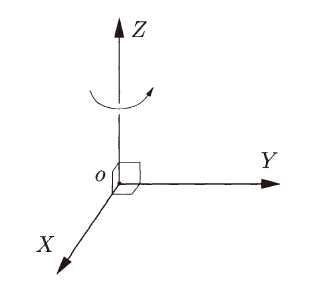因此，叉积自然地定义了矢量$u$$3×3$斜对称矩阵$\hat{u}$之间的映射。通过检查，该陈述的反过来显然是正确的，因为我们可以容易地识别与每个相关联的三维矢量。 $3 \times 3$斜对称矩阵（仅从式（1）中提出$u_1,u_2,u_3$）。

### 反对称矩阵

矩阵$M\in \mathbb{R}^{3\times 3}$是斜对称的，当且仅当对某个$u\in \mathbb{R}^3$使得满足$M=\hat{u}$时。

因此，向量空间$\mathbb{R}^3$和所有斜对称3×3矩阵的空间(被称为$\mathfrak{so}(3)$是同构的（即，存在保持向量空间结构的一对一映射）。 同构是所谓的hat算子(hat operator):

$\land : \mathbb{R} \rightarrow \mathfrak{so}(3):u\mapsto \hat{v}$

它的逆映射，称为vee算子，从斜对称矩阵$\hat{u}$中提取向量$u$的分量，由下式给出：

$\vee : \mathfrak{so}(3)\rightarrow \mathbb{R}^3;\hat{u}\mapsto \hat{u}^{\vee}=u$

## 刚体运动

考虑在相机前移动的物体。 为了描述其运动，原则上应该指定物体上每个单点的轨迹，例如，通过将点的坐标指定为时间$X(t)$的函数。 幸运的是，对于刚性物体，我们不需要指定每个点的运动。 正如我们稍后将看到的，仅指定一个（而不是每个）点的运动以及连接到该点的三个坐标轴的运动就足够了，如图2所示。 原因在于，对于每个刚性物体，物体上任何两点之间的距离不随物体移动而随时间变化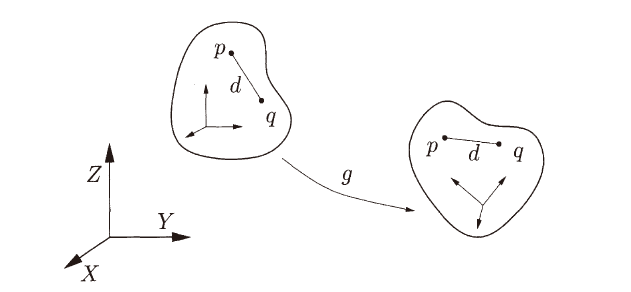因此，如果$X(t)$$Y(t)$分别是对象上任意两个点$p$$q$的坐标，则它们之间的距离是恒定的：

$||X(t)-Y(t)||\equiv {\rm constant},\forall t\in \mathbb{R}\tag{2}$

刚体运动（或刚体变换）是一系映射，描述刚性物体上每个点的坐标在满足式（2）时如何随时间变化。 我们用这个映射来表示

$g(t):\mathbb{R}^3\rightarrow \mathbb{R}^3;X \mapsto g(t)(X),t\in[0,T]$

如果不是看着移动物体的整个连续路径，而是集中在它的初始和最终位姿之间的映射上，我们有一个刚体位移，用

$g:\mathbb{R}^3\rightarrow \mathbb{R}^3;X \mapsto g(X)$

除了变换点的坐标之外，$g$还引起向量的变换。 假设$v$是由坐标$v = Y-X$的两个点$p$$q$定义的向量; 然后，在变换$g$的作用下之后，我们获得一个新的向量

$u=g_*(v)\dot=g(Y)-g(X)$

由于$g$保留了点之间的距离，我们得到对于所有自由向量$v\in \mathbb{R}^3$,都有$g_*(v)=||v||$

保留距离的映射称为欧氏变换。 在3-D空间中，所有欧氏变换的集合由$E(3)$表示。 请注意，保持点之间的距离不足以表征在空间中移动的刚性物体。 实际上，存在保留距离的变换，但它们不是物理上可实现的。 例如，映射

$f:[X_1,X_2,X_3]^T \mapsto [X_1,X_2,-X_3]^T$

保留距离但不保留方向。 它对应于XY平面中的点作为双面镜的反射。 为了排除这种映射，我们要求任何刚体运动除了保持距离外，还要保留方向。 也就是说，除了保留向量的范数之外，它还必须保留它们的叉积。 由刚体运动引起的映射或变换称为特殊欧氏变换。 “特殊”一词表示变换是保向的。

### 刚体运动或特殊欧氏变换

映射$g:\mathbb{R^3 \rightarrow R^3}$是一个刚体运动或特殊欧氏变换，仅当它保留任何两个向量的范数和叉积，

1. 范数：$||g_*(v)||=||v||,\forall v\in \mathbb{R}^3$
2. 叉积：$g_*(u)\times g_*(v) =g_*(u\times v),\forall u,v \in \mathbb{R}^3$

在刚体运动的上述定义中，保持矢量之间的角度并不是显而易见的。 但是，内积$<\cdot,\cdot>$可以通过极化恒等式（Polarization Identity）用范数$||\cdot||$表示。

$<u,v>=\frac{1}{4}(||u+v||^2-||u-v||^2)$

由于$||u+v||=||g_*(u)||+||g_*(v)||$，可以得出结论，对于任何刚体运动$g$

$<u,v>=<g_*(u),g_*(v)>,\forall u,v \in \mathbb{R}^3$

换句话说，刚体运动也可以定义为保留内积和叉积的运动。

#### 例子：三重积和体积

从刚体运动的定义可以看出，它还保留了三个向量中所谓的三重积（Triple Product）：

$<g_*(u),g_*(v)\times g_*(w)>=<u,v\times w>,\forall u,v,w \in \mathbb{R}^3$

由于三重积对应于三个矢量所构成的平行六面体的体积，因此刚体运动也保持了体积。

这些属性如何帮助我们简洁地描述刚体运动？ 通过刚体运动保持距离和方向的事实意味着各个点不能相对于彼此移动。 因此，刚体运动可以通过身体上所选点的运动和附着于该点的坐标系的旋转来描述。 为了看到这一点，我们通过将笛卡尔坐标系附加到刚体上的某个点来表示刚体的配置，并且我们将跟踪该坐标系相对于固定世界（参考）系的运动。

为此，考虑一个坐标系，其轴由三个正交向量$e_1,e_2,e_3\in \mathbb{R}^3$给出; 也就是说，它们满足

$e_i^Te_j=\delta_{ij}\dot=\begin{cases}\delta_{ij}=1 &for \quad i= j \\\delta_{ij}=0 &for \quad i \neq j \end{cases}$

通常，对向量是排序的以至于形成右手坐标系：$e_1\times e_2=e_3$。 然后，在刚体运动$g$后，我们有：

$g_*(e_i)^Tg_*(e_j)=\delta_{ij},g_*(e_1)\times g_*(e_2)=g_*(e_3)$

也就是说，得到的三个矢量仍然形成右手正交坐标系。因此，刚性物体总是可以与右手正交坐标系相关联，并且其刚体运动可以完全由这种坐标系的运动来指定，我们称之为物体坐标系。

也就是说，得到三个向量$g_*(e_1),g_*(e_2),g_*(e_3)$仍然形成右手正交坐标系。因此，刚性对象总是可以与右手正交坐标系相关联，我们将其称为对象坐标系或体坐标系，其刚体运动可以完全由这种坐标系的运动来指定。

在图3中，我们示出了一个物体，在这种情况下是一个相机，相对于预先选择的世界参考系$W:(X,Y,Z)$移动。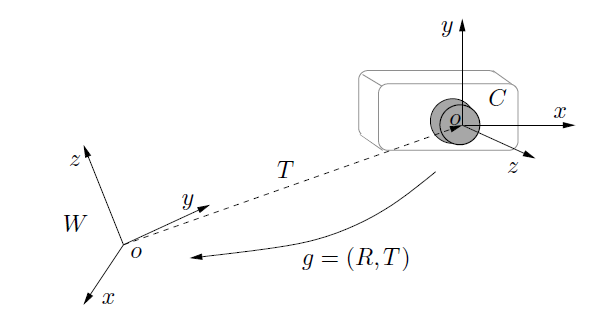为了指定相机相对于世界坐标系$W$的配置，可以在相机上选择固定点$o$并且在其上附接体坐标系，在这种情况下称为相机坐标系，$C=(x,y,z)$。 相机移动时，相机坐标系也会随相机移动。 然后，摄像机的配置由两个部分决定：

1. 世界坐标系的原点$o$与相机坐标系的原点$o$之间的向量$g(o)$，称为“平移”部分，用$T$表示;
2. 相对于具有坐标轴$（X，Y，Z）$的固定世界坐标系$W$，具有坐标轴$（x，y，z）$的相机坐标系$C$的相对方向，称为“旋转”部分，由$R$表示。

在我们考虑的问题中，世界参考系及其原点$o$没有明确的选择。 因此，我们可以选择要附加到摄像机的世界坐标系并指定场景相对于该坐标系的平移和旋转（只要它是刚性的），或者我们可以将世界坐标系附加到场景并指定相对于该坐标系的相机运动。 重要的是场景和相机之间的相对运动; 从几何学的角度来看，世界参考坐标系系的选择是任意的。

如果我们可以将刚性物体（例如，相机）从一个地方移动到另一个地方，我们当然可以反转动作并将其恢复到原始位置。 同样，我们可以组合几个动作来生成一个新的动作。 粗略地说，这种可逆性和组成的性质可以用“群”的概念在数学上进行表征。

我们很快就会看到，刚体运动集确实是一个群，即所谓的特殊欧氏群。 然而，在我们给出一个显式表示并将其用于计算之前，群的抽象概念是没有用的。 在接下来将重点研究如何用矩阵表示刚体运动。 更具体地说，我们将证明任何刚体运动都可以表示为$4 \times 4$矩阵。 为简单起见，我们从刚体运动的旋转分量开始。

## 旋转运动及其表示

### 旋转的正交矩阵表示

假设我们有一个刚性物体绕固定点$o\in \mathbb{E}$旋转。 我们如何描述相对于选定坐标系的方向，比如$W？$。 不失一般性，我们可能总是假设世界坐标系的起源是旋转中心$o$。 如果不是这种情况，只需将原点转换为点$o$即可。 我们现在将另一个坐标系（例如$C$）附加到旋转对象，比如相机，其原点也在$o$处。 这两个坐标系之间的关系如图4所示。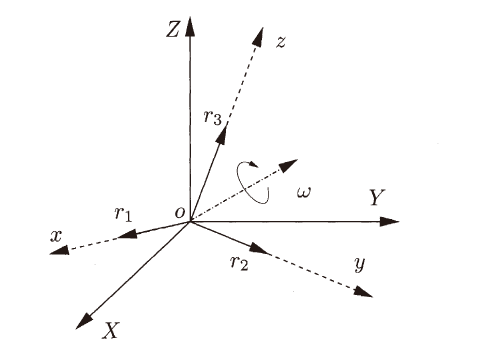坐标系$C$相对于坐标系$W$的配置（或“方向”）由相对于世界坐标系$W$的三个正交矢量$r_1=g_*(e_1),r_2=g_*(e_2),r_3=g_*(e_3)\in \mathbb{R}^3$的坐标确定,如图4所示。 三个矢量$r_1,r_2,r_3$分别简单是沿着相机坐标系$C$上的轴$x,y,z$的单位矢量。然后，旋转物体的配置完全由$3×3$矩阵确定

$R_{wc}\dot=[r_1,r_2,r_3]\in \mathbb{R}^{3\times3}$

其中$r_1,r_2,r_3$按顺序堆叠为三列。 由于$r_1,r_2,r_3$形成正交坐标系，因此遵循这一点

$r_i^Tr_j=\delta_{ij}\dot=\begin{cases}1 &for \quad i= j ,\\0 &for \quad i \neq j ,\end{cases}\forall i,j\in \{1,2,3\}$

这可以用矩阵形式写成

$R_{wc}^TR_{wc}=R_{wc}R_{wc}^T=I$

满足上述恒等式的任何矩阵称为正交矩阵。 从上面的定义得出，正交矩阵的逆是简单的转置：$R_{wc}^{-1}=R_{wc}^T$。由于$r_1,r_2,r_3$形成右手坐标系，我们还有进一步的条件，即$R_{wc}$的行列式必须是$\pm 1$。 因此，$R_{wc}$是一个特殊正交矩阵，与之前一样，“特殊”一词表示它是保向的。$\mathbb{R}^{3\times 3}$中所有这些特殊正交矩阵的空间通常表示为

$SO(3)\dot=\{R\in \mathbb{R}^{3\times 3}|RR^T=I,\det(R)=\pm 1\}$

一般的，$3\times3$特殊正交矩阵被称为旋转矩阵。 可以证实$SO(3)$在矩阵乘法下满足群的所有四个公理。简单说旋转矩阵乘法符合分配律，结合律。其逆是其转置也是旋转矩阵，单位元素是$I$即不旋转。

因此，空间$SO(3)$也被称为$\mathbb{R}^{3\times 3}$的特殊正交群，或简称为旋转群。 直接从定义中可以看出，旋转确实保留了向量的内积和叉积。

### 旋转矩阵

表示围绕$Z$轴旋转角度$\theta$的矩阵是

$R_Z(\theta)=\begin{bmatrix}cos(\theta)&-sin(\theta)&0\\sin(\theta)& cos(\theta) &0\\0 &0&1\end{bmatrix}$

回到图4，每个旋转矩阵$R_{wc}\in SO(3)$表示物体围绕点$o$旋转。 除此之外，$R_{wc}$还表示从坐标系$C$到坐标系$W$的实际坐标变换的矩阵。

为了看到这一点，假设对于给定的点$p\in \mathbb{E}^3$，其相对于坐标系$W$的坐标是 $X_w = [X_{1w}, X_{2w}, X_{3w}]^T \in \mathbb{R}^3$。 自$r_1,r_2,r_3$形成$\mathbb{R}^3$的基（是坐标系$C$的轴），$X_w$也可以表示为这三个矢量的线性组合，例如$X_w=X_{1c}r_1+X_{2c}r_2+X_{3c}r_3$,其中 $X_c = [X_{1c}, X_{2c}, X_{3c}]^T \in \mathbb{R}^3$。 显然， $X_c = [X_{1c}, X_{2c}, X_{3c}]^T$是相对于坐标系$C$的相同点$p$的坐标。因此，我们有：

$X_w=X_{1c}r_1+X_{2c}r_2+X_{3c}r_3=R_{wc}X_c$

在该等式中，矩阵$R_{wc}$将点$p$相对于坐标系$C$的坐标$X_c$变换为相对于坐标系$W$的坐标$X_w$。由于$R_{wc}$是旋转矩阵，因此其逆是简单的转置：

$X_c=R^{-1}_{wc}X_w=R_{wc}^TX_w$

也就是说，旋转的逆变换也是旋转; 我们称之为$R_{cw}$:

$R_{cw}=R_{wc}^{-1}=R_{wc}^T$

然后可以将物体的连续旋转描述为空间$SO(3)$中的轨迹$R(t):t\mapsto SO(3)$。 当起始时间不是$t = 0$时，时间$t_2$和时间$t_1$之间的相对运动将表示为$R(t_2,t_1)​$。由旋转群的结合律可以得到

$R(t_2,t_0)=R(t_2,t_1)R(t_1,t_0),\forall t_0<t_1<t_2\in \mathbb{R}$

然后，对于旋转摄像机，固定的3D点$p$的世界坐标$X_w$通过以下方式变换为相对于摄像机坐标系C的坐标：

$X_c(t)=R_{cw}X_w$

或者，如果相对于具有坐标$X_c$的相机坐标系的点$p$，则其作为$t$的函数在其世界坐标$X_w(t)$由下式给出：

$X_w(t)=R_{wc}(t)X_c$

### $SO(3)$上的指数坐标（映射）

到目前为止，我们已经证明$\mathbb{E}^3$中的旋转刚体运动可以用$3\times 3$旋转矩阵$R\in SO(3)$表示。 在我们到目前为止的矩阵表示中，每个旋转矩阵R由其$3\times 3=9$个元素描述和确定。 但是，这9个元素不是自由参数 - 它们必须满足约束$R^TR=I$。这实际上对9个元素施加了6个独立约束(两两正交约束)。 因此，旋转矩阵空间$SO(3)$的维度仅为$3$，并且9中的6个参数实际上是多余的。 稍后我们将为旋转矩阵引入一些表示（或参数化）。

给定曲线$R(t):\mathbb{R} \rightarrow SO(3)$ 描述连续旋转运动，旋转必须满足以下约束：

$R(t)R^T(t)=I$

计算上述方程相对于时间$t$的导数，注意到右侧是一个常数矩阵，我们得到：

$\frac{d}{dt}(R(t)R^T(t))=\dot{R}(t)R^T(t)+R(t)\dot{R}^T(t)=0 \Rightarrow \dot{R}(t)R^T(t) = -(\dot{R}(t)R^T(t))^T$

得到的等式反映了矩阵$\dot{R}(t)R^T(t)\in \mathbb{R}^{3\times 3}$是斜对称矩阵的事实。 然后，正如我们在前面叉积时所说的，必存在一个向量，比如$\omega(t)\in \mathbb{R}^3$，使得：

$\dot{R}(t)R^T(t)=\hat{\omega}(t)$

将$R(t)$乘以等式两端右侧得到：

$\dot{R}(t)=\hat{\omega}(t)R(t)$

根据上面的等式，如果对于$t=t_0$，如果$R(t_0)=I$，则我们有$\dot{R}(t_0)=\hat{\omega}(t_0)$。 因此，在单位矩阵$I$周围，斜对称矩阵给出了旋转矩阵的一阶近似（一阶泰勒展开）：

$R(t_0+dt) \approx I+\hat{\omega}(t_0)dt$

所有斜对称矩阵的空间都表示为

$\mathfrak{so}(3)\dot=\{\hat{\omega}\in \mathbb{R}^{3\times 3}|\omega\in \mathbb{R}^3\}$

它也被称为旋转群$SO(3)$在原点（单位矩阵，identity）处的切空间

$SO(3)$被称为李群，$\mathfrak{so}(3)$被称为李代数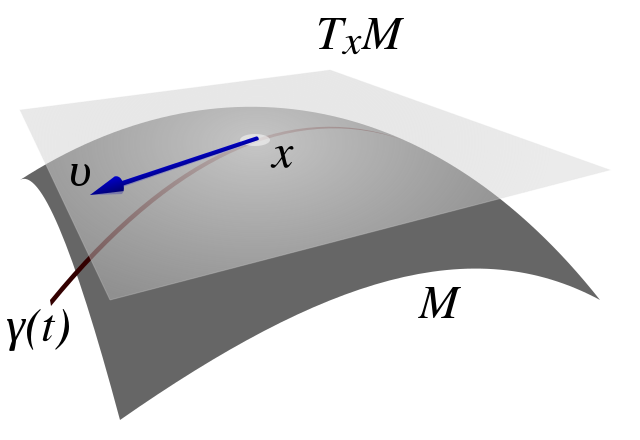如果$R(t)$不是原点，则$R(t)$处的切空间简单地通过$R(t)$右乘$\mathfrak{so}(3)$变到$R(t)$$\dot{R}(t)=\hat{\omega}(t)R(t)$。 这也表明$SO(3)$的元素仅取决于三个参数$(\omega_1,\omega_2,\omega_3)$

在理解了它的局部近似之后，我们现在将使用这些知识来获得旋转矩阵的表示。 我们假设矩阵$\hat{\omega}$$\dot{R}(t)=\hat{\omega}(t)R(t)$是常数：

$\dot{R}(t)=\hat{\omega}R(t)$

在上面的等式中，$\hat{\omega}$可以解释为以下线性常微分方程（ODE）的状态转移矩阵（state transition matrix）：

$\dot{x}(t)=\hat{\omega}x(t),x(t)\in \mathbb{R}^3$

然后立即验证上述ODE的解是由下式给出的：

$x(t)=e^{\hat{\omega}t}x(0)\tag{3}$

其中$e^{\hat{\omega}t}$是矩阵指数函数：

$e^{\hat{\omega}t}=\sum\limits_{n=0}^{\infty}\frac{(\hat{\omega}t)^n}{n!}=I+\hat{\omega}t+\frac{(\hat{\omega}t)^2}{2!}+\cdots+\frac{(\hat{\omega}t)^n}{n!}+\cdots\tag{4}$

$e^{\hat{\omega}t}$也被记为$\exp (\hat{\omega}t)$。由于式子（3）的ODE解唯一性，并假设$R(0)=I$作为初始条件，我们有：

$R(t)=e^{\hat{\omega}t}\tag{5}$

为了证明矩阵$e^{\hat{\omega}t}$确实是一个旋转矩阵，可以直接从矩阵指数的定义中看出：

$(e^{\hat{\omega}t})^{-1}=e^{-\hat{\omega}t}=e^{\hat{\omega}^Tt}=(e^{\hat{\omega}t})^T$

因此$(e^{\hat{\omega}t})^Te^{\hat{\omega}t}=I$。仍然表明$\det (e^{\dot{\omega}t})=\pm 1$

等式（5）的物理解释是：如果$||\omega||=1$，则$R(t)=e^{\hat{\omega}t}$只是围绕旋转轴$\omega\in \mathbb{R}^3$旋转$t$弧度。

因此，矩阵指数（等式4）确实定义了从空间$\mathfrak{so}(3)$$SO(3)$的映射，即所谓的指数映射：

$\exp:\mathfrak{so}(3)\rightarrow SO(3)$

$\hat{\omega}\in \mathfrak{so}(3)\mapsto e^{\hat{\omega}}\in SO(3)$

注意，我们通过假设等式（5）中的$\omega(t)$是常数来获得等式（4）的。 但情况并非总是如此。 因此，这里自然会出现一个问题：每个旋转矩阵$R\in SO(3)$能否以等式（5）中的指数形式表示？ 答案是肯定的。

### $SO(3)$中的李对数

对于任何旋转矩阵$R\in SO(3)$，存在$\omega\in \mathbb{R}^3$，使得$R=\exp(\hat{\omega})$。 这样的元素用$\hat{\omega}=\log (R)$表示(指数映射的逆)。

$j$即对于任何$R\in SO(3)$，存在（不一定是唯一的）$\omega\in \mathbb{R}^3,||\omega||=1$$t\in \mathbb{R}$使得$R(t)=e^{\hat{\omega}t}$

该定理的证明是通过构造来证明。

如果旋转矩阵$R$给出为：

$R=\begin{bmatrix}r_{11}&r_{12}&r_{13}\\r_{21}&r_{22}&r_{23}\\r_{31}&r_{32}&r_{33}\end{bmatrix}$

相应的$t$$\omega$ 由下给出：

$t=\cos^{-1}\left(\frac{{\rm trace}(R)-1}{2}\right),\omega=\frac{1}{2 \sin(t)}\begin{pmatrix}r_{32}-r_{23}\\ r_{13}-r_{31}\\r_{21}-r_{12} \end{pmatrix}$

该定理的重要性在于，可以通过围绕某个轴旋转一定角度来实现任何旋转矩阵

然而，该定理仅保证了从$\mathfrak{so}(3)$$SO(3)$的指数映射的可靠性。 不幸的是，这个映射不是单射的，因此不是一对一的。 在我们引入所谓的罗德里格斯公式计算$R(t)=e^{\hat{\omega}t}$的公式后，这将变得清晰。

现在我们现在知道如何计算给定旋转矩阵$R\in SO(3)$的指数坐标$\omega$。 另一方面，给定$\omega$，我们如何有效地计算相应的旋转矩阵$R=e^{\hat{\omega}}$？ 人们当然可以使用等式5来求解。 然而，以下定理大大简化了计算：

### 罗德里格斯公式（Rodrigues’ formula）及证明

给定$\omega\in \mathbb{R}^3$，其中$||w||=1$而且$t\in \mathbb{R}$,则矩阵指数$R(t)=e^{\hat{\omega}t}$可以通过下面的公式给出：

$e^{\hat{\omega}t}=I+\hat{\omega}\sin(t)+\hat{\omega}^2(1-\cos(t))$

这是直接验证$\hat{\omega}$的幂！ 可以通过以下两个公式来减少幂的次方：

$\hat{\omega}^2=\hat{\omega}\hat{\omega}^T-I$

$\hat{\omega}^3=-\hat{\omega}$

因此，等式4的指数级数可以简化为：

$e^{\hat{\omega}t}=I+\underbrace{(t-\frac{t^3}{3!}+\frac{t^5}{5!}-\cdots)}_\text{sin(t)} \hat{\omega}+\underbrace{(\frac{t^2}{2!}-\frac{t^4}{4!}+\frac{t^6}{6!}-\cdots)}_\text{1-cos(t)}\hat{\omega}^2$

所以得到

$e^{\hat{\omega}t}=I+\hat{\omega}\sin(t)+\hat{\omega}^2(1-\cos(t))$

该证明摘自维基百科。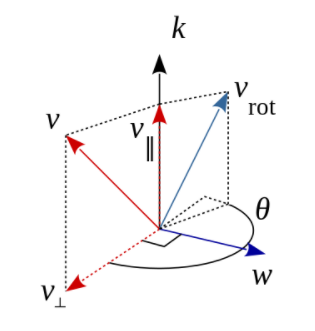如上图所示，$k$是单位向量，它定义了旋转轴，而$v$是任意的一个向量，但是围绕$k$轴旋转$\theta$角得到$v_{rot}$.

向量$v$被分解为与$k$轴平行$v_{\|}$和与$k$垂直的$v_{\perp}$分量，由内积可以得到这两个分量与$v$的关系为$v=v_{\|}+v_{\perp}$

与$k$轴平行的分量$v_{\|}$表示为：

$v_{\|}=(v\cdot k)k$

$v\cdot k$$v$在向量$k$上的投影长度，然后乘以单位向量$k$就得到$v$$k$方向为分量的$v_{\|}$

上式被称为$v$$k$的向量投影。

而垂直于$k$的分量为$v_{\perp}$，通过前两个式子可以得到：

$v_{\perp}=v-v_{\|}=v-(k\cdot v)k=-k\times (k\times v)$

称为来自$k$$v$的向量拒绝（ vector rejection ）。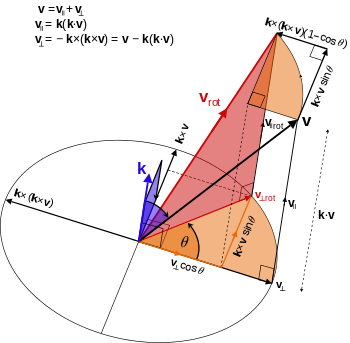从上图来说明，$k \times v$可以看作是$v_{\perp}$关于$k$逆时针旋转$90^\circ$的副本，所以它们大小相等，但是方向垂直【你得注意到$k$是单位向量的这个假设】。同样，向量$k\times (k\times v)$$v_{\perp}$关于$k$逆时针旋转$180^\circ$的一个副本，所以$k\times (k\times v)$$v_{\perp}$在大小上相等，但方向相反（因为他们互为相反数，所以是减号）。

三重积展开式建立了平行分量和垂直分量间的联系：

$a\times (b\times c)=(a\cdot c)b-(a\cdot b)c,\forall a,b,c$

平行于轴的分量在旋转下不会改变幅度和方向，所以$v_{\| rot}=v_{\|}$

只有垂直分量会改变方向，但会保持其大小（仅旋转）。

$|v_{\perp rot}=|v_{\perp}|$

$v_{\perp rot}=\cos \theta v_{\perp} + \sin \theta \ k\times v_{\perp}$

由于$k$$v_{\|}$是平行的，所以叉积为零，即

$k\times v_{\|}$

所以可以得到

$k\times v_{\perp}=k\times v-k\times v_{\|}=k\times v$

因此

$v_{\perp rot}=\cos \theta \ v_{\perp} + \sin \theta \ k\times v$

这种选择是正确的，这是因为向量$v_{\perp}$$k\times v$具有相同的长度，而且$k\times v$$v_{\perp}$绕着$k$轴逆时针旋转$90^\circ$得到的。

使用三角函数正弦和余弦对$v_{\perp}$$k\times v$进行适当缩放给出旋转的垂直分量。

旋转分量形式类似于笛卡尔基的2D平面极坐标$(r,\theta)$中给出的径向向量

$r=r \cos \theta \ e_x+r \sin \theta \ e_y$

其中$e_x,e_y$则是坐标轴方向指向的单位向量。

那么完整的选择矢量就有了表达：

\begin{aligned} v_{rot} &=v_{\|}+\cos \theta \ v_{\perp} + \sin \theta \ k\times v \\ &=v_{\|}+\cos \theta \ (v-v_{\|}) +\sin \theta \ k\times v \\ &= \cos \theta \ v+(1-\cos \theta)v_{\|}+ \sin \theta \ k\times v\\&= \cos \theta \ v+ (1-\cos \theta)(k\cdot v)k+ \sin \theta \ k\times v \end{aligned}

以上是旋转向量的推导，然后将$v$$k\times v$表示为列矩阵，叉积可以表示为矩阵乘积(之前的博文和本博文之前有描述叉积，帽运算)：

$k\times v = \begin{bmatrix}(k\times v)_x\\(k\times v)_y\\(k\times v)_z\end{bmatrix}=\begin{bmatrix}k_yv_z-k_zv_y\\k_zv_x-k_xv_z\\k_xv_y-k_yv_x\end{bmatrix}=\begin{bmatrix}0&-k_z&k_y\\k_z&0&-k_x\\-k_y&k_x&-0\end{bmatrix}\begin{bmatrix}v_x\\v_y\\v_z\end{bmatrix}$

令$K$表示单位向量$k$的叉积矩阵

$K=\begin{bmatrix}0&-k_z&k_y\\k_z&0&-k_x\\-k_y&k_x&-0\end{bmatrix}$

所以可得到下面等式

$k\times v=Kv$

对于任何向量$v$（实际上，$K$是这个性质唯一的矩阵，它具有特征值$0$$\pm 1$）。

迭代右边叉积相当于左乘叉积矩阵：

$K(Kv)=K^2v=k\times (k\times v)$

由于$k$是单位向量，所以具有单位2-范数。

所以在矩阵语言上，我们有矩阵公式形式的旋转公式：

$v_{rot}=v+(\sin \theta)Kv+(1-\cos \theta)K^2v,||K||_2=1$

上述形式的由来是这样的。

我们知道$v_{rot} = \cos \theta \ v+ (1-\cos \theta)(k\cdot v)k+ \sin \theta \ k\times v$,而且$v_{\perp}=v-v_{\|}=v-(k\cdot v)k=-k\times (k\times v)$

所以我们可以得到

$(k\cdot v)k=v+k\times (k\times v)$

所以

\begin{aligned} v_{rot} &= \cos \theta \ v+ (1-\cos \theta)(k\cdot v)k+ \sin \theta \ k\times v \\ &= \cos \theta \ v+ (1-\cos \theta)（v+k\times (k\times v)）+ \sin \theta \ k\times v\\ &=\cos \theta \ v+ (1-\cos \theta)v+(1-\cos \theta) k\times (k\times v)+ \sin \theta \ k\times v\\ &= v+(1-\cos \theta) \underbrace{k\times (k\times v)}_{K^2v}+ \sin \theta \ \underbrace{k\times v}_{Kv} \\ &=v+(\sin \theta)Kv+(1-\cos \theta)K^2v\end{aligned}

值得注意的是，这个公式的主要项现在是1。

将$v$因式分解可以得到紧凑的表达式：

$v_{rot}=Rv$

其中

$R=I+(\sin \theta)K+(1-cos(\theta))K^2$

是围绕$k$轴旋转为$\theta$的旋转矩阵，$I$$3\times 3$的单位矩阵。其中

$K=\hat{k}$

$K^2=\hat{k}\hat{k}=kk^T-I$

所以

\begin{aligned}R&= I+\sin \theta \ \hat{k} +(1-\cos \theta)(kk^T-I)\\&= \cos \theta I+\sin \theta \ \hat{k} +(1-\cos \theta) kk^T\\&=e^{\hat{k} \theta}\end{aligned}

证明完毕。

#### 罗德里格斯公式中元素与旋转矩阵的关系及证明

下面我们证明，由罗德里格斯的公式：

$R=e^{\hat{\omega}t}=I+\hat{\omega}\sin(t)+\hat{\omega}^2(1-\cos(t))$

如何得到下面的等式？

$t=\cos^{-1}\left(\frac{{\rm trace}(R)-1}{2}\right),\omega=\frac{1}{2 \sin(t)}\begin{pmatrix}r_{32}-r_{23}\\ r_{13}-r_{31}\\r_{21}-r_{12} \end{pmatrix}$

首先，证明：

$t=\cos^{-1}\left(\frac{{\rm trace}(R)-1}{2}\right)$

我们知道

$R=e^{\hat{\omega}t}=I+\hat{\omega}\sin(t)+\hat{\omega}^2(1-\cos(t))$

$\Leftrightarrow R=\cos\theta \ I+\hat{\omega}\sin(t)+(1-\cos(t))\omega\omega^T$

两边对其求迹【常数的迹还是常数】，可以得到

\begin{aligned}{\rm trace}(R) &= \cos(t) \ \underbrace{ {\rm trace}(I)}_{=3}+\sin(t) \ \underbrace{ {\rm trace}(\hat{w}) }_{=0}+(1-\cos(t))\ \underbrace{ {\rm trace} (\omega\omega^T) }_{=1}\\&=3\cos(t) +(1-\cos(t))\\&=1+2\cos(t) \end{aligned}

这就能得到:

$t=\cos^{-1}\left(\frac{{\rm trace}(R)-1}{2}\right)=\arccos\left(\frac{{\rm trace}(R)-1}{2}\right)$

对于$\omega$,求解可能较复杂,我们用最愚蠢的方式证明，假设

$\omega=\begin{bmatrix}\omega_1\\\omega_2\\\omega_3\end{bmatrix}$

则：

$\hat{\omega}=\begin{bmatrix}0&-\omega_3&\omega_2\\\omega_3&0&-\omega_1\\-\omega_2&\omega_1&-0\end{bmatrix}$

$R=\begin{bmatrix}r_{11}&r_{12}&r_{13}\\r_{21}&r_{22}&r_{23}\\r_{31}&r_{32}&r_{33}\end{bmatrix}$

由于

$\hat{\omega}^T=-\hat{\omega}$

所以

$(\hat{w}^2)^T=(\hat{\omega}^T)^2=\hat{\omega}^2$

所以我们让$R=I+\hat{\omega}\sin(t)+\hat{\omega}^2(1-\cos(t))$两边都取转置得到：

$R^T=I-\hat{\omega}\sin(t)+\hat{\omega}^2(1-\cos(t))$

所以很容易得到

$\hat{\omega}\sin (t)=\frac{R-R^T}{2}$

由于

$\hat{\omega}\sin(t)=\begin{bmatrix}0&-\omega_3&\omega_2\\\omega_3&0&-\omega_1\\-\omega_2&\omega_1&-0\end{bmatrix}\sin(t)$

而且

$R-R^T=\begin{bmatrix}r_{11}&r_{12}&r_{13}\\r_{21}&r_{22}&r_{23}\\r_{31}&r_{32}&r_{33}\end{bmatrix}-\begin{bmatrix}r_{11}&r_{21}&r_{31}\\r_{12}&r_{22}&r_{32}\\r_{13}&r_{23}&r_{33}\end{bmatrix}$

$\Leftrightarrow R-R^T=\begin{bmatrix}0&r_{12}-r_{21}&r_{13}-r_{31}\\r_{21}-r_{12}&0&r_{23}-r_{32}\\r_{31}-r_{13}&r_{32}-r_{23}&0\end{bmatrix}$

然后两者联立得到

$\hat{\omega}=\begin{bmatrix}0&-\omega_3&\omega_2\\\omega_3&0&-\omega_1\\-\omega_2&\omega_1&-0\end{bmatrix}=\frac{1}{2\sin(t)}\begin{bmatrix}0&r_{12}-r_{21}&r_{13}-r_{31}\\r_{21}-r_{12}&0&r_{23}-r_{32}\\r_{31}-r_{13}&r_{32}-r_{23}&0\end{bmatrix}$

故而得到

$\omega_1=r_{32}-r_{23},\omega_2=r_{13}-r_{31},\omega_3=r_{21}-r_{12}$